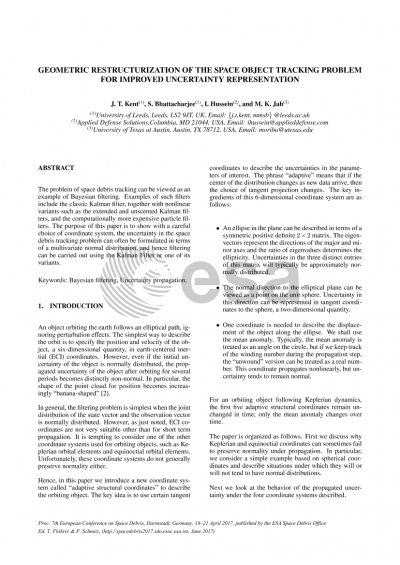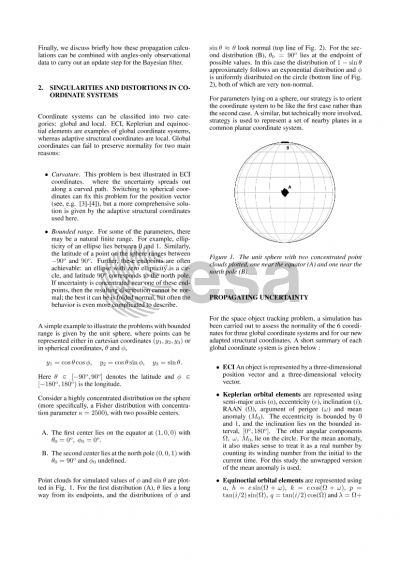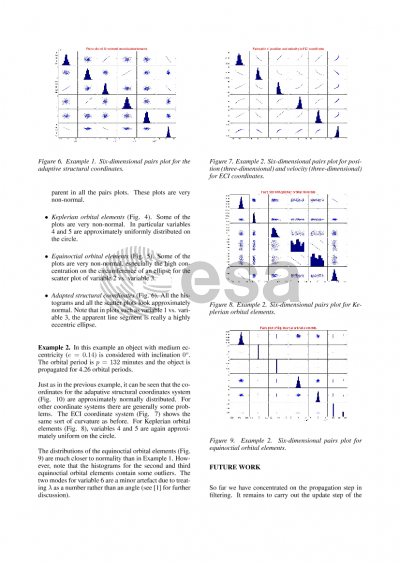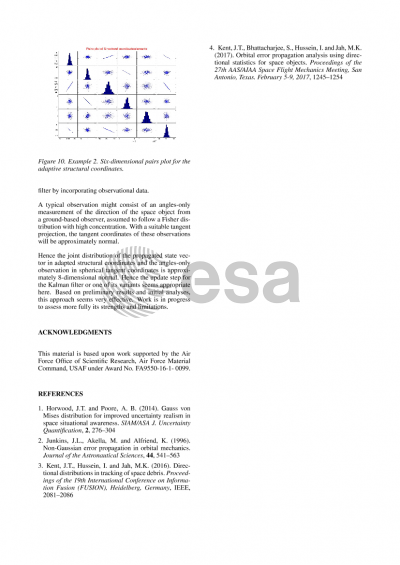# Geometric restructurization of the space object tracking problem for improved uncertainty representation

John T. Kent1,Shambo Bhattacharjee1,Islam Hussein2,Moriba K. Jah3
University of Leeds1Applied Defense Solutions2University of Arizona3

## Document details

Publishing year2017 PublisherESA Space Debris Office Publishing typeConference Name of conference7th European Conference on Space Debris
Pagesn/a Volume
7
Issue
1
Editors
T. Flohrer, F. Schmitz
Download paper## Abstract

The problem of space debris tracking can be viewed as an example of Bayesian filtering. Examples of such filters include the classic Kalman filter, together with nonlinear variants such as the extended and unscented Kalman filters, and the computationally more expensive particle filters. The purpose of this paper is to show with a careful choice of coordinate system, the uncertainty in the space debris tracking problem can often be formulated in terms of a multivariate normal distribution, and hence filtering can be carried out using the Unscented Kalman Filter(UKF).

Up to perturbation effects, an object in orbit around the earth follows an elliptical path. The simplest way to describe the orbit is in terms of earth-centered inertial (ECI) coordinates, namely the position and velocity of the object. However, even if the initial uncertainty of the state is normally distributed, following the orbital path for several periods into the future leads to a propagated uncertainty which is distinctly non-normal . The other ingredient in the filtering problem is a set of measurements, taken here to be a set of directions at successive times seen by an observer on the surface of the earth.

In general, the filtering problem is simplest when the joint distribution of the state vector and the observation vector is normally distributed. To achieve this goal, we introduce a new set of "adaptive structural coordinates" to describe the orbiting object and the measurements of the object. The key ingredients of this coordinate system are:

(a) Five coordinates to describe an ellipse in three dimensions (two for the normal direction to the plane of the ellipse, and three to describe an ellipse in the plane). Under the propagation step, these coordinates remain fixed.

(b) One coordinate to describe the position of the object along the ellipse, namely the true anomaly. Typically, it is treated as an angle on the circle, but if we keep track of the winding number during the propagation step, its "unwound" version can be treated as a real number. This coordinate propagates nonlinearly, but still roughly preserves normality in many cases.

(c) Two coordinates to describe an observation, i.e. the direction of the space object from the observer's perspective on the earth's surface.

For random vectors on the unit sphere (i.e. the normal vector to the plane of orbit, and the directional vector of observations), we suggest using "adaptive" tangent plane coordinates. That is, if a distribution on the sphere is concentrated about a fixed unit direction, then we use tangent coordinates centered at that unit direction to get an approximate normal distribution. The phrase "adaptive" means that if the center of the distribution changes as new data arrive, then the choice of tangent projection changes.

The adaptive structural coordinate system given here is similar in spirit to a coordinate system proposed by Horwood and Poore , but the coordinates here seem to do better at giving normality. In addition, the "Gauss-von Mises" distribution, which Horwood and Poore create to accommodate the wrapped true anomaly as an angular variable, reduces in certain circumstances to a 6-dimensional multivariate normal distribution when the unwrapped true anomaly is used instead.

Empirically, we have found that if we start with a concentrated normal distribution for the state vector in either ECI coordinates or adaptive structural coordinates, then the joint distribution of the propagated state vector and the measurement direction is also approximately normal in adaptive structural coordinates; this property definitely does not hold in ECI coordinates. We have looked at the propagation of point clouds in adaptive structural coordinates with a variety of choices for the propagation time, and for orbits with varying amounts of eccentricity.

There are still a few issues before a Bayesian filter can be implemented. First, the unwrapped true anomaly has been treated as a Euclidean variable in the state vector, but it is the wrapped value which is relevant when comparing the propagated state vector to the observation direction. This problem can be tackled by mixture modelling. And second, exact or approximate forms for the 8-dimensional covariance matrix of the propagated state plus observation are needed. Based on preliminary results, adaptive structural system of coordinates lends itself naturally to the UKF.

References

1. Junkins, J.L., Akella, M. and Alfriend, K. (1996) Non-Gaussian error propagation in orbital mechanics. Journal of the Astronautical Sciences, 44, 541-563.

2. Horwood, J T and Poore, A B (2014) Gauss von Mises Distribution for Improved Uncertainty Realism in Space Situational Awareness. SIAM/ASA J. Uncertainty Quantification, 2, 276-304.

## Preview